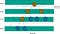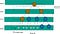# My mental model for Heap Structure

A heap is a binary tree holding a distinct relation between every parent and their nodes — a parent’s value (or key) is always less than (min-heap) or greater than (max-heap) their children.Stacked, Balanced, Perfection. www.Studio51Film.com Instagram @sturgeon_imagery

Here’s a quick takeaway if you don’t want to read the whole thing.

A (min)heap data-structure (a.k.a Priority queue) is model as a binary tree where each parent node’s value (or key) is always less than (or equal to) their children.

They’re conveniently implemented using an array.

For a parent node at index ’n’, you’ll find their children at (2n + 1) and (2n + 2) indices.

For a node at index ’n’, you’ll find its parent at index Floor(n — 1)/2

For adding an element, we add at the end of the array and bubble- up the array(beginning).

For deletion, we replace the first element with the last element and bubble-down the array(end).

We shall be explaining on “min-heap” though the same concept can be equivalently applied to “max-heap” as well.

The term “heap” doesn’t reflect anything, at least to me, on the nature of this tree (read — binary tree) and if you see the photo above, that’s the real-world concept of a heap — a random collection of things placed haphazardly on top of each other. However, the structure that we are discussing here maintains a strict ordering between the items and therefore doesn’t resemble this mental modal whatsoever shape or form.

On top of this (the difference between the real-concept and abstract-concept), almost all of the discussion on heap structure started right away explaining with Binary Tree structure and immediately implemented using the Array structure. What is missing though is the explanation of why we model it as one structure (binary tree) but implemented with another structure (i.e. Array in this case). In order to understand this concern, we need to dive deeper and look again into the heap property and the algorithms to add and delete items from it.

Before that, you may ask me — how do I map (or hook) the concept of the heap into my mental model? The way I remember is that heap is nothing but a fancy name of “Priority Queue” — a queue of things where each thing carries a weight called “priority” with it. In such queue, low priority element is served before an element with high priority (the reverse is true for max-heap)

With that interpretation, let’s jump into the analysis.

Heap property — Every parent node (read as node’s value) must be smaller than or equal to its children (left & right child), In other words, every parent node must not be greater than its left and right sub-tree(s).

How would we satisfy this property using Binary Tree in all operations, notably the addition and deletion of items?

For binary search tree(BST), as the parent node is always between its left child and right child, insertion and deletion are easily done using a well-defined BST algorithm.

For heap binary tree, let’s start a thought analysis with a single node P. That is also a heap. No problem. Let’s assume there’re new items M and N coming in, and imagine that they’re smaller than P. On what basis would we decide that which one would be a left or right child? We can’t just decide randomly there. Again, if they are larger than P or if anyone is larger than P, how would we decide the final arrangement without breaking the heap property. We can’t arrange them randomly because it may break the heap property at some insertion or/and deletion operations.

Nonetheless, there’re ways to implement this ( https://stackoverflow.com/questions/18241192/implement-heap-using-a-binary-tree) arrangement using binary tree itself, but we’ll not go into the details of them (perhaps another article to analyze their complexity with that of Array implementation).

We will be looking at how Array might solve this issue of arranging parents and their child nodes (rather beautifully yet intuitively) though maintaining the heap property.

One key thing that you might already see in Array but not there in Tree is that — Array elements are uniquely ordered from 0, then 1, 2, and so on…And that’s not the case with Tree and therefore we’ve different traversals of a tree structure (Post Order, In order, Pre Order, Breadth-first, Depth-first, etc). And most notably, an array index P is always less than any subsequent indices that come after it. Sounds similar to the heap property?

Let’s draw binary tree representation of a min-heap structure and we’ll see how it can be mapped to Array easily.

The above binary tree can also be drawn as below. Why not? A tree could have weird branches!😁

And imagine, we map each node (of the binary tree) to an array element as shown below -

Voila! we mapped the binary tree into an array representation. And interesting thing is that an array element can be treated as a parent node and all its children (which is on its right side, not necessarily adjacent one) will always be greater than or equal to its value. We now satisfied the heap property.

Also, for an array element (read as a node in a binary heap) at array index “n”, you’ll find its children (left and right child) at (2n + 1) and (2n + 2) indices respectively. And for a node at array index “n”, its parent would be found at Floor(n — 1)/2 as well.

Well, you may be thinking about how I magically derive those children's locations (2n + 1) & (2n + 2) for a given parent location at index n.

Here’s the missing point in the earlier definition of Heap. Heap is also a complete binary tree. All the nodes of the tree are arranged to completely fill all levels (think of as a breadth-first/level-traversal way) except possibly the lowest level which in that case needs to be filled from the left up to a point.

That’s quite a mouthful 😳 . Let’ see what I mean by the diagram 😁(A complete binary tree)

If you check the above tree, you’ll observe that the nodes are filling up level-by-level (starting from root) from left to right. So, it’s filling up with 1 node (level 0), 2 nodes (level 1), 4 nodes (level 2), and next it should 8 maximum nodes (level 3) that we can fill up. So maximum nodes at a level n are 2ⁿ, assuming level starts from 0. Whenever a level has fewer nodes than its maximum and if we start filling the nodes from the left, that’s what the complete binary tree is referring to (as you can see in the diagram).

However, the diagram below, though it may satisfy the heap property is not a complete binary tree as we have empty slots on the left (at level 3). This binary heap cannot be mapped to an array using the formula (2n + 1) and (2n + 2)(An incomplete binary tree)

So, to cut the story short here’s the complete definition of the binary heap, rephrase again: A heap is a complete binary tree holding a distinct relation between every parent and their nodes — a parent’s value (or key) is always less than their children. Each node of the tree can be mapped to an array index using the formula — A parent at index n will have its children at indices (2n + 1) and (2n + 2) respectively.

In other words, only when the binary heap is almost complete, then will we able to lay down the children's locations in an array, using the above formula.

Finally, coming to the addition and deletion of items in Binary heap (refer TLDR above), the algorithm demands to insert an element at the end or beginning of an array and that is constant time, O(1) complexity in an array, which is not so in a binary tree.

This explains the reason why we describe Heap structure as a Binary Tree but usually implement rather as an Array structure.

I know this topic is on the longer side. We would also like to discuss the a) complexity of heap structure, b) implementation in Javascript and also c) two primary applications of heap data structure — 1) Merging K-Sorted arrays and 2) Djikstra’s shortest path algorithm

We shall continue discussing the remaining topic next time. So, stay tuned!